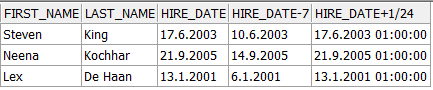# Oracle SQL Tutorials – Chapter 3 (Part 2 of 3)

NUMERIC FUNCTIONS

ROUND

• Let’s try some different usages of the ROUND function.
```SELECT

ROUND (45.923),

ROUND (45.943, 1),

ROUND (45.924, 2),

ROUND (44.923, -1),

ROUND (55.923, -2)

FROM DUAL;```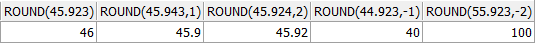TRUNC

• Let’s try some different usages of the TRUNC function.
```SELECT

TRUNC (45.923),

TRUNC (45.943, 1),

TRUNC (45.953, 2),

TRUNC (45.923, -1),

TRUNC (45.923, -2)

FROM DUAL;```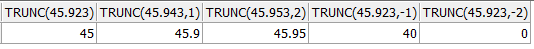FLOOR

• Here’s the usage of the FLOOR function.
```SELECT FLOOR(46.993)

FROM DUAL;```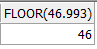MOD

• Here’s the usage of the MOD function
```SELECT MOD(1245,10)

FROM dual;```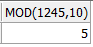SIGN

• The usage of the SIGN is like below.
```SELECT SIGN(15),

SIGN(-15)

FROM dual;```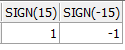DATE FUNCTIONS

• Let’s see how to use MONTHS_BETWEEN, ADD_MONTHS, NEXT_DAY, LAST_DAY, ROUND, TRUNC functions.
```SELECT

MONTHS_BETWEEN (TO_DATE ('01.08.2013', 'dd.mm.yyyy'),

TO_DATE ('01.01.2013', 'dd.mm.yyyy'))"Months Between",

SYSDATE "Today",

NEXT_DAY (SYSDATE, 'MONDAY') "Next Monday",

LAST_DAY (SYSDATE) "Last Day of this month",

ROUND (SYSDATE - 60, 'MONTH') r1,

ROUND (SYSDATE, 'YEAR') r2,

TRUNC (SYSDATE, 'MONTH') t1,

TRUNC (SYSDATE, 'YEAR') t2

FROM DUAL;```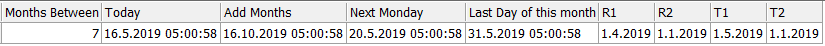ARITHMETIC OPERATIONS WITH DATES

Date + Number = Date : It adds days to date, as entered value.

Date – Number = Date : It subtracts days from date , as entered value.

Date – Date = Number of days : It gives the number of days between two dates.

Date + ( Number/24) = Date : It adds hours as entered value to the date.

• Let’s see how to use these operations.
```SELECT

first_name,

last_name,

hire_date,

hire_date - 7,

hire_date + 1 / 24

FROM hr.employees

WHERE department_id = 90;```